# Civil Engineering - Irrigation

### Exercise :: Irrigation - Section 3

21.

V and R are the regime mean velocity and hydraulic mean depth respectively in metres. Lacey's silt factor f is

 A.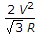B.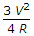C.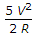D.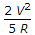Explanation:

No answer description available for this question. Let us discuss.

22.

Pick up the correct statement from the following

 A. Escapes are essential safety valves in a canal system B. The capacity of escapes should not be less than the capacity of the canal at its location C. The escapes must lead the surplus water to natural drainages D. The escapes are aligned to take advantage of contours of lower values E. All the above.

Explanation:

No answer description available for this question. Let us discuss.

23.

According to Kennedy, the critical velocity (V0) in metres in a channel is the mean velocity which keeps the channel free from silting or scouring. Its value is given by (where m is critical velocity ratio and D is the depth of the channel).

 A.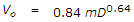B.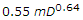C.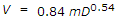D.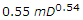Explanation:

No answer description available for this question. Let us discuss.

24.

According to Lacey, depth of scour in a river depends upon the straightness of the reach. If D is the depth of scour in regime flow in a right angled bend, it is

 A. 1.25 D B. 1.50 D C. 1.75 D D. 2.00 D

Explanation:

No answer description available for this question. Let us discuss.

25.

The width of a dowla is generally kept between 30 to 60 cm and its height above the road level should invariably be more than

 A. 10 cm B. 20 cm C. 30 cm D. 40 cm